﻿ Voltage and current phase angle frequency measurement method_Guang Zhou ZhengNeng Electronic Technology Co., Ltd. ﻿
• 中文
• Weibo
• WeChatApplication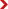Field applicationTechnical articles
Technical articles

### Voltage and current phase angle frequency measurement method

Click：       Source：Zhengneng Technology

Guangzhou Zhengneng ES2010 double clamp phase voltammeter can measure AC voltage, current, phase and frequency, jaw size: φ7.5mm, phase range: 0.0~360°, current range: 0.0mA~20.0A, voltage range: 0.00 V ~ 600V, the biggest feature is that you can measure the frequency. The following describes how to use ES2010 to measure the voltage, current, phase angle, and frequency of a low-voltage power distribution cabinet.

Measured low voltage distribution cabinet on-site image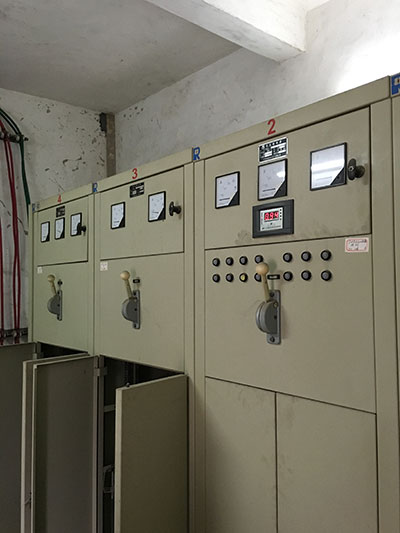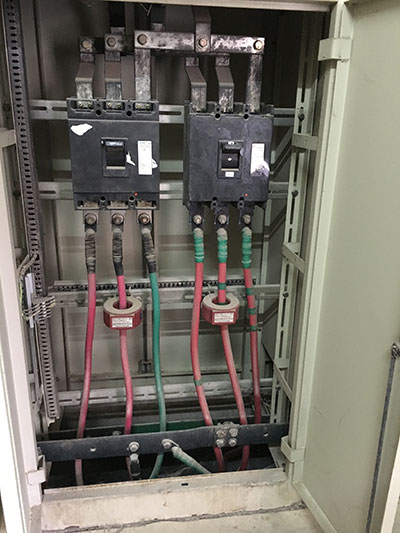ES2010 double clamp phase voltammeter standard accessories are: 1 host, 1 instrument box, 2 current clamps, 4 test leads (2 red and black), 1 battery 9V alkaline battery, manual, certificate 1 setTake out the ES2010 double clamp phase voltammeter, measure the voltage as shown below, and the wiring has other wiring diagrams.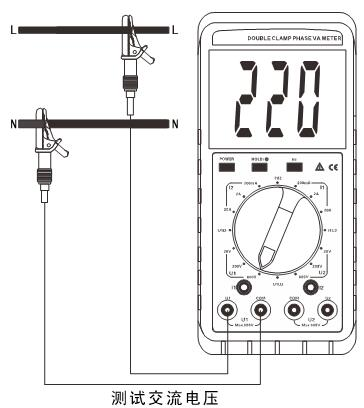When measuring the voltage, first estimate the approximate range, then rotate to a comparable gear. This voltage should be around 400V, so rotate to 600V, 200V-600V will be measured with this file.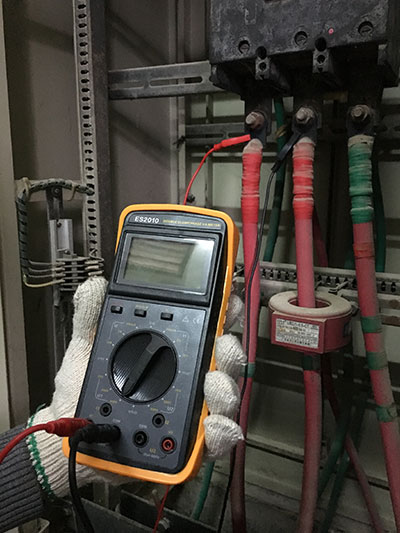Press the POWER button to turn it on, and the voltage at this time is 402V.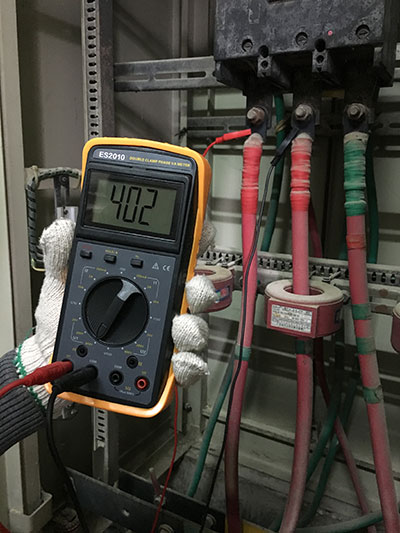At this point, the phase of Ib and Uab is measured, and the phase of I1U2 is reached, and the phase is 179.1 degrees.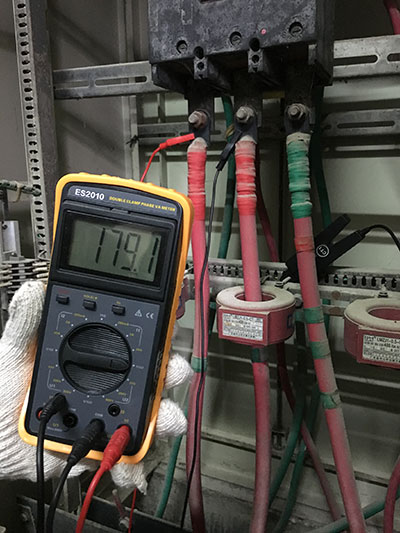The ES2010 meter can measure the frequency and press the HZ button to get the frequency. When the rotary switch is turned to the current or voltage position of U1 or U2, the current frequency can be measured by press Hz. The frequency at this time is 50HZ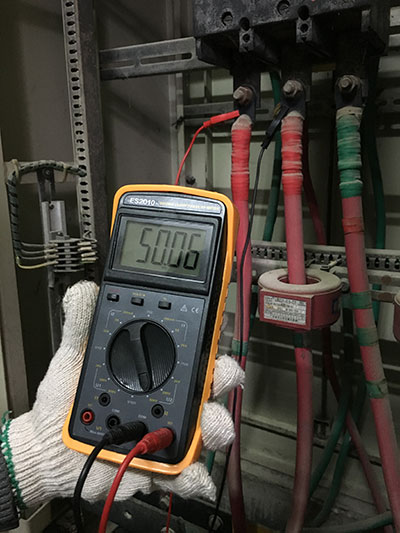Press the CT1 current clamp into the I1 port to measure the current.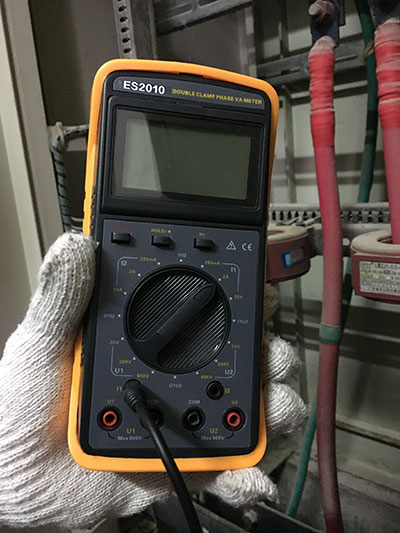Rotate to the current gear and estimate the current. The current at this time is 1.26A.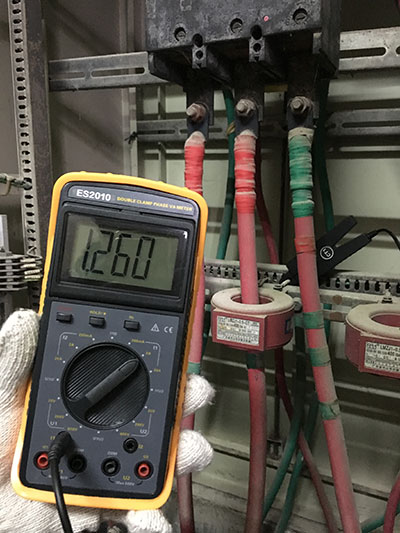After the measurement is completed, press POWER to shut down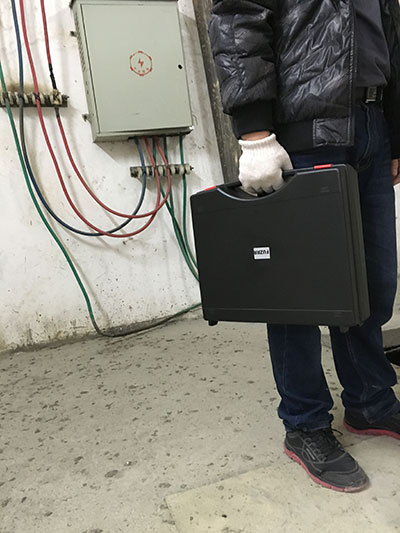The above is an example of parameters such as the voltage and current phase angle frequency of the low-voltage power distribution cabinet measured by Guangzhou Zhengneng ES2010 Double Clamp Phase Voltammeter